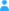Sign in

|

Dependencies in Dataset #1387

during ESMVal mission

Entries (observed variables)

Information about observed variables of the current dataset.
No. Name
01 cub (cubname)
02 time [unspecified unit]
03 altitude [unspecified unit]
04 observer altitude (km)
05 observer azimuth [degree]
06 longitude [degree_east]
07 latitude [degree_north]
08 HNO3 volume mixing ratio (ppbv)
09 precision of HNO3 volume mixing ratio (ppbv)
10 accuracy of HNO3 volume mixing ratio (ppbv)
11 vertical resolution (FWHM) of HNO3 volume mixing ratio (km)
12 measurement contribution of HNO3 volume mixing ratio 
13 H2O volume mixing ratio (ppmv)
14 precision of H2O volume mixing ratio (ppmv)
15 accuracy of H2O volume mixing ratio (ppmv)
16 vertical resolution (FWHM) of H2O volume mixing ratio (km)
17 measurement contribution of H2O volume mixing ratio 
18 O3 volume mixing ratio (ppmv)
19 precision of O3 volume mixing ratio (ppmv)
20 accuracy of O3 volume mixing ratio (ppmv)
21 vertical resolution (FWHM) of O3 volume mixing ratio (km)
22 measurement contribution of O3 volume mixing ratio 
23 pressure (hPa)
24 precision of pressure volume mixing ratio (hPa)
25 accuracy of pressure volume mixing ratio (hPa)
26 vertical resolution (FWHM) of pressure volume mixing ratio (km)
27 measurement contribution of pressure volume mixing ratio 
28 temperature (K)
29 precision of temperature volume mixing ratio (K)
30 accuracy of temperature volume mixing ratio (K)
31 vertical resolution (FWHM) of temperature volume mixing ratio (km)
32 measurement contribution of temperature volume mixing ratio 
A virtual entry: n_height given by 108 constant values
B virtual entry: n_cublen given by 15 constant values
C virtual entry: n_prof given by 257 constant values

Dependencies of the observed variables

The interactive table provides a visualization of the dependencies of this dataset's entries (variables) which will hopefully assist you to understand the datasets structure.

Most datasets are simple, e.g. like 10 entries depending on 1 entry. But there are other datasets which are far more complex; with relationships between various entries and even reversed dependencies.

Warning: It works only with JavaScript enabled in your browser.

Direction of relationships

Entry A (=depends on)

Explanation

Enable Javascript and you'll see the dependencies of this dataset explained in this box while hovering over the table on the left.

-->